# CAT Questions on Circles

0
3624

## CAT Questions on Circles:

CAT Geometry questions and answers on Circles. Important solved problems on geometry circles topic based on previous year papers.

Try checking out previous year papers of CAT to know about the level of questions that appear in the exam.

Question 1:

A circle having a diameter of 16 cm is cut by a chord of length 8cm. Find the length of the smaller arc of the circle.

a) $$\frac{2\pi}{3}$$
b) $$\frac{16\pi}{3}$$
c) $$\frac{4\pi}{3}$$
d) $$\frac{8\pi}{3}$$

Question 2:

A semicircle is inscribed in a triangle with sides 22, 26 and 40 as shown in the figure. What is the diameter of the semicircle?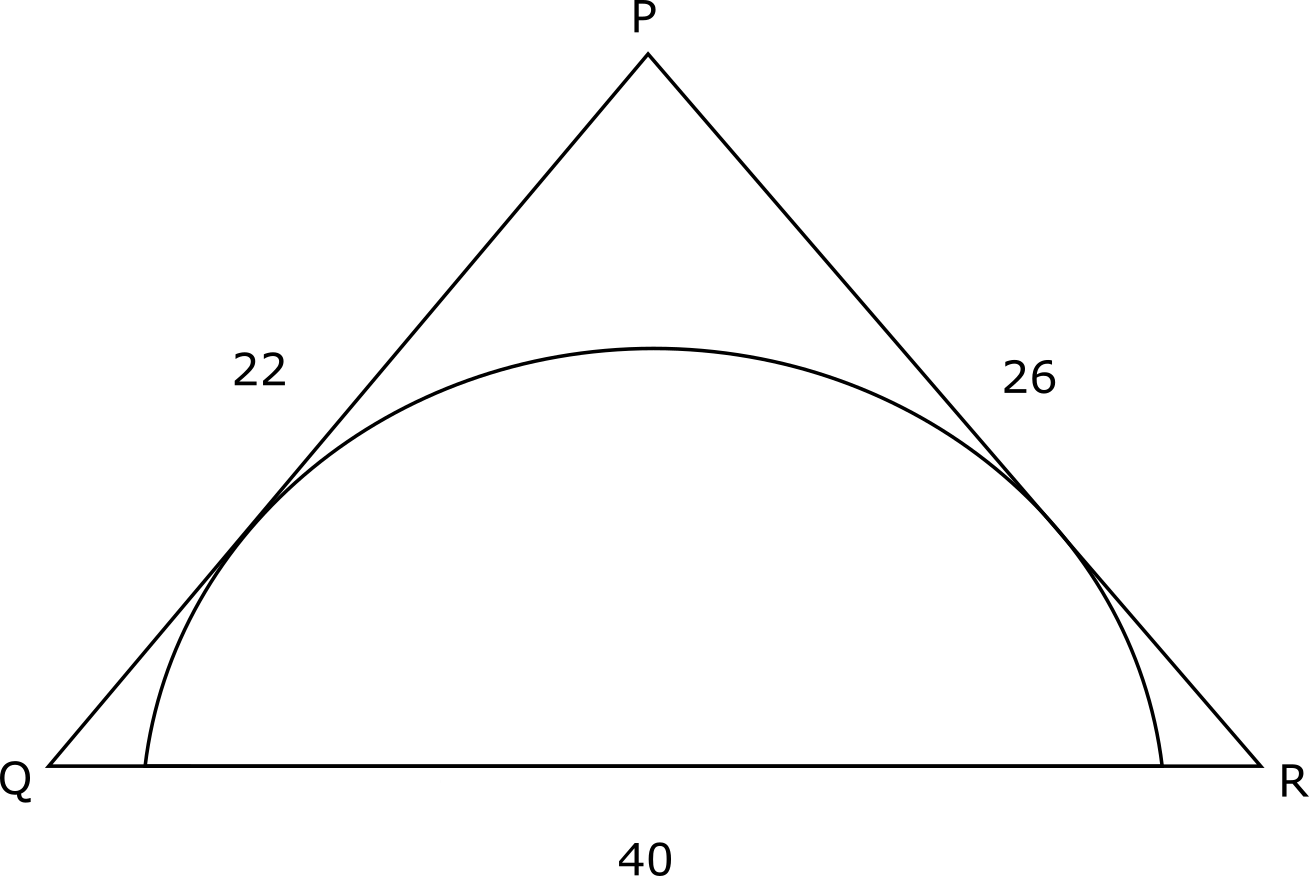a) 11 cm
b) 12 cm
c) 22 cm
d) 24 cm

Question 3:
Tangents are drawn from the points (6,8) to the circle $$x^2+y^2=36$$. Find the angle between the two tangents approximately.

a) $$64^{\circ}$$
b) $$74^{\circ}$$
c) $$84^{\circ}$$
d) $$94^{\circ}$$

Solutions: (1 to 3)

Refer to the following figure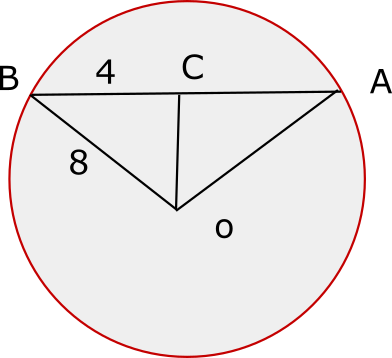In triangle COB
sin COB = 4/8 = 1/2
COB = 30 degrees
BOA = 30*2 = 60 degrees
Length of BOA =$$\frac{60}{360}*2\pi 8 =\frac{8\pi}{3}$$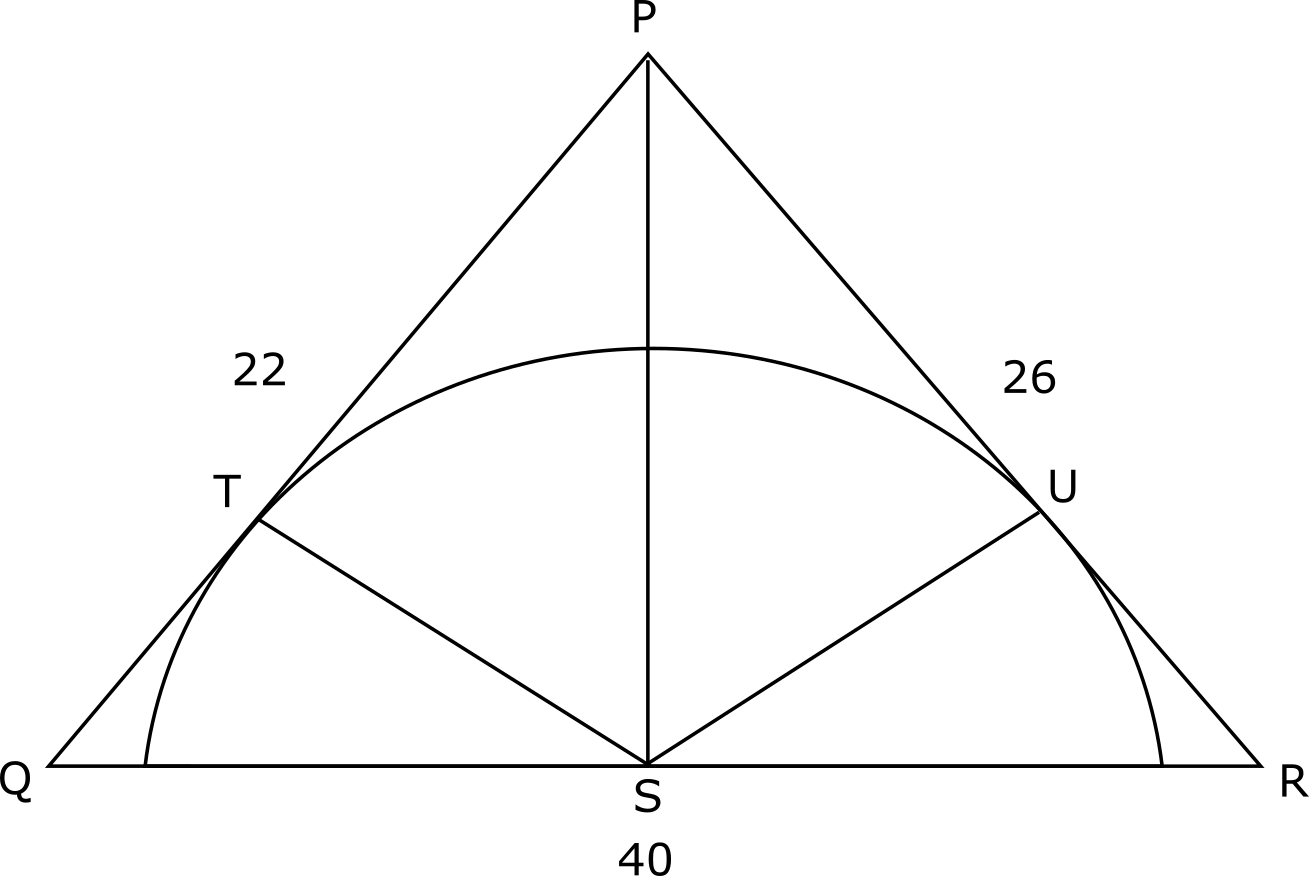Draw the perpendiculars to PQ and PR as shown. The perpendiculars are the radii of the semicircle.

Let’s look at triangles PTS and PUS. PT = PU. ST = SU. PS is the common side. So both the triangles are similar to each other.
So, angle SPT = angle SPU.

Using angle bisector theorem, $$\frac{PQ}{PR}=\frac{QS}{SR}$$
Also, SR = 40-QS.
Solving, we get QS = $$\frac{55}{3}$$

Triangle QST is a right triangle and applying cosine rule in triangle PQR, cos Q = $$\frac{4}{5}$$
=> sin Q = $$\frac{3}{5}$$

sin Q = $$\frac{ST}{QS}$$ => ST = 11.

Hence the diameter is 22 cm.

Enroll to any CAT Course – Use coupon “KICKSTART25”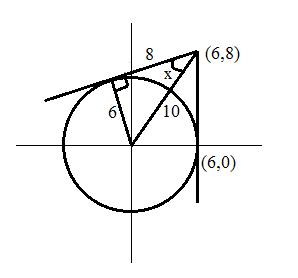Let the angle between the tangents be 2x.
The radius of the circle is 6 and the center is (0,0).
=> Distance betweenthe center and point (6,8) is $$\sqrt{6^2+8^2}$$ = 10
=> Length of the tangent = $$\sqrt{10^2-6^2}$$ = 8
$$\sin x$$ = 6/10 = 0.6
$$\sin 30^{\circ}$$ = 0.5 and $$\sin 45^{\circ}$$ = $$\frac{1}{\sqrt{2}}$$ = 0.7
=> x must be around $$35^{\circ}$$ to $$38^{\circ}$$
=> 2x must be around $$70^{\circ}$$ to $$76^{\circ}$$
Among the options, option B is the closest answer.

Question 4:

In the figure given below AB = 9, AC = 15 and BC = 12. A circle is drawn with BE as a diameter such that AB and AC are tangents to it. Find the value of diameter of the circle.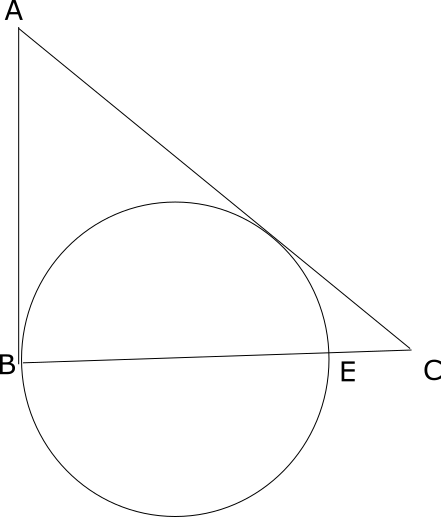Answer: 9

Solution:

Consider the following figure, F is the center of the circle.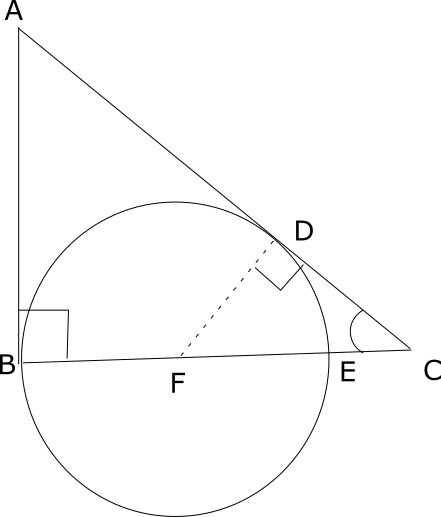In triangles ABC and FDC.
Angle ABC= Angle FDC=90
Angle ACB s common. Thus, both are similar.
=> 9/15=r/12-r
=> r=4.5
Thus, diameter=9cm

Question 5:

A circle touches all the three sides of the triangle as shown in the given figure at points P, Q and R respectively. If Angle RPQ is 50 degrees, find the measure of angle BAC (in degrees)?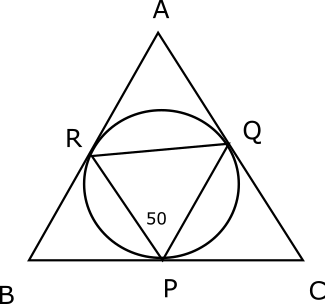Solution:
Let O be the centre of the circle. Join OQ and OR. Since angle subtended by the arc at the centre is double the angle subtended at any other point,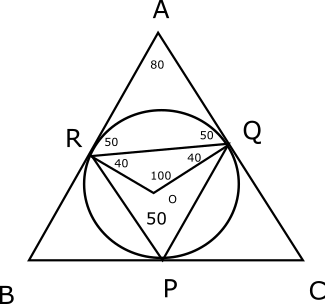Angle = ROQ = 2*50 = 100 degrees
Since OR = OQ
Angle ORQ = Angle OQR = (180-100)/2 = 40 degrees
Since line drawn from the centre to the point of contact is perpendicular to the tangent,
Angle ARQ = Angle AQR = 90-40 = 50 degrees
Angle RAQ = 180-50-50 = 80 degrees.

CAT Circle questions on Geometry is useful as questions are asked based on Circles in CAT exam.